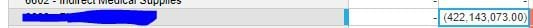# Odd Division OutputWould someone explain why Anaplan is giving me a number 5x larger than the number I am dividing when my calculation takes the first number (4,221.43) and divides it by 0? I would assume that it would be 0 or a NaN. Not something as large as the last number.Tagged:

•Definitely a little odd

If you round the source (in the source module), do you still get the same result

I think it looks like you are using ratio as the summary method. I wonder if that is the cause

Is the result odd for detailed members and/or totalsl?

David